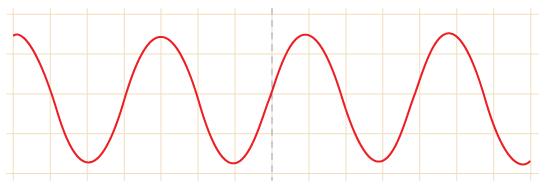In this article we are going to talk about the Computer Basics : The Bits and The Bytes.Computers are used to process data. Computers works on instructions to process data. They do it electrically inside CPU(central processing unit). Computer don’t really understand the language that a human mind can understand.There processing capabilities lies within bits and bytes. So we now know that a PC understand in “Bits”.

### The Bits

A Bit is an abbreviation for Binary Digit(0 or 1). Each and Every “1” or a “0” is defined a “BIT”. Example:

1  —–> 1 bit

0 —–> 1 bit

10101 ——> 5 bit

10101110——-> 8 bit

This was easy!

The binary number system consists of only 0’s and 1’s to define any letter or digit. For an instance, We all know that to use decimal system to define a digit such as 5 or 45, in decimal number system, we use 0-9 digits to define a combination of numbers. Binary means “2”. 2 is defined as the base of binary systems.

Now lets learn how to define a decimal number in binary format.We need to use power of base 2 to convert simple numbers into binary such as 20,21,22..etc to define a digit.
```20= 1 21= 2 22= 4 23= 8 . . .```

 Number in Decimal System. Number in Binary System. . 8 4 2 1 0 0 0 0 0 1 0 0 0 1 2 0 0 1 0 3 0 0 1 1 4 0 1 0 0 5 0 1 0 1 6 0 1 1 0 7 0 1 1 1 8 1 0 0 0 9 1 0 0 1 10 1 0 1 0 11 1 0 1 1 12 1 1 0 0 13 1 1 0 1 14 1 1 1 0 15 1 1 1 1

So this way we add those binary powers and get decimal system numbers. You might be wondering ,the data that we enter in a computer is beyond 1 or 2 digits, So how a computer compute all those numbers together to produce a letter or a combination of numbers.To go on with that explanation lets first know little something about analog and digital data.

#### Analog Data:

The basic form a human can interpret data is in the analog form. That means the data that we receive while doing daily chores such as listening music or talking to someone or writing something or singing something, graphics, movies etc. The data have its own varied form that means each data received is continuously changing and a human mind can easily interpret this wave form.#### Digital Data:

Computers is a electric unit. Computers can only deal with electrically switched data that means on or off just same as a switch. Likewise, It can only deal with binary ‘0’ or ‘1’. These switches in a computer is in the form of transistors. A computer has billions of transistors so as to easily interpret data.Now If a computer could define our daily chores from analog to digital data then it would define it as a huge combination of ‘0’s and ‘1’s. The word processing is the basic of data processing. Every letter or digit in our keyboard should be defined digitally in the computer so that computer can understand. Also, there are other symbols too such as @#!\$%^&*()_+{}:”<>. These are defined by streams of bits.

### The Bytes

A stream of 8 bits is called 1 byte. A Byte is a 8 digit number in the binary form, So to define the number of different bytes using 8 binary digit we can calculate 2 x 2 x 2 x 2 x 2 x 2 x 2 x 2 = 256. 256 different bytes defining 256 different letters and digits and characters. So this 256 different combination of binary is the System!These 256 different bytes slots is defined clearly by ASCII table. This means that a word such as “pineapple” consists of 9 letters. This word would be converted into 9 bytes after digitizing and will occupies 9 byte of RAM and 9 bytes in hard disk if saved!

What is ASCII?

ASCII means American Standard Code for Information Interchange. It is an industry standard, which assigns letters,
numbers, and other characters within the 256 slots available in the 8 bit code.
The ASCII table is divided in 3 sections:

• Non printable system codes between 0 and 31.
• “Lower ASCII” between 32 and 127. This part of the table originates from older, American ADP systems, which
worked on 7 bit character tables. Foreign letters, like Ø and Ü were not available then.
• “Higher ASCII” between 128 and 255. This part is programmable, in that we can exchange characters, based on which language we want to write in. Foreign letters are placed in this part.

To See Entire ASCII table Visit : http://www.ascii-code.com/

Lets have an Example for the whole scenario:

If we type something on keyboard then streams of bit will be sent to CPU, this way if the stream of bits are

00110110001100100010000000100100

This stream of 32 bits will be divided into 4 bytes and then be compared with the ASCII table hence the bits represent:  62 \$

This will provide you the basic idea of working of computers.Though the scenario inside a computer is complex, this article will provide basic interpretation.This site uses Akismet to reduce spam. Learn how your comment data is processed.